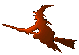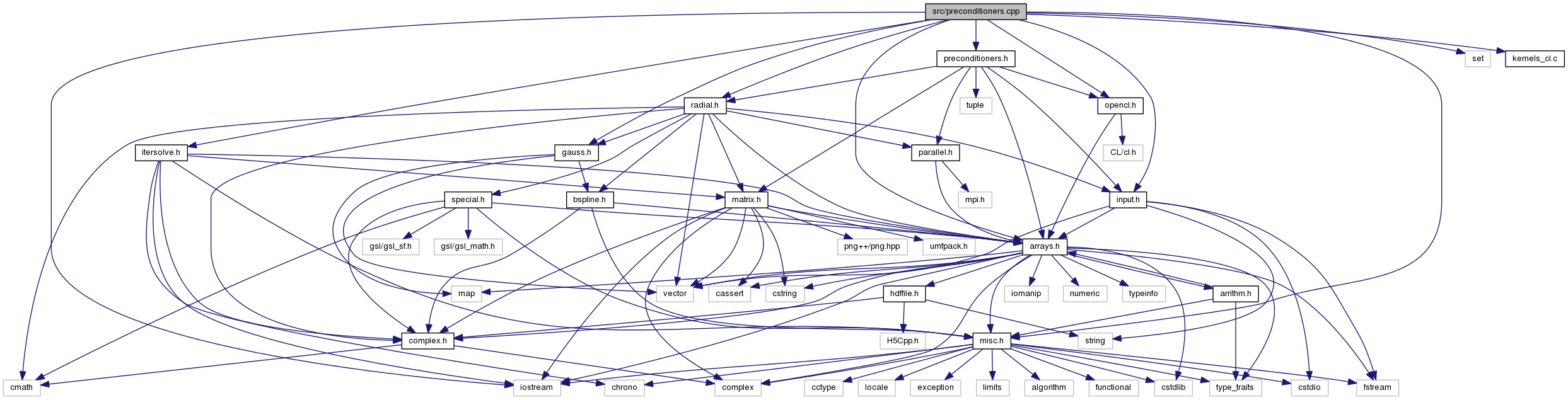Hex  1.0 Hydrogen-electron collision solver
preconditioners.cpp File Reference
#include <iostream>
#include <set>
#include "arrays.h"
#include "gauss.h"
#include "input.h"
#include "itersolve.h"
#include "misc.h"
#include "preconditioners.h"
#include "radial.h"
#include "opencl.h"
#include "kernels_cl.c"
Include dependency graph for preconditioners.cpp:## Macros

#define THRESHOLD   1e-5

## Functions

cArray IC (cArrayView const &A, lArrayView const &I, lArrayView const &P)

SymDiaMatrix DIC (SymDiaMatrix const &A)
DIC preconditioner. More...

SymDiaMatrix SSOR (SymDiaMatrix const &A)
SSOR preconditioner. More...

void zgelsd_ (int *M, int *N, int *NRHS, Complex *A, int *LDA, Complex *B, int *LDB, double *S, double *RCOND, int *RANK, Complex *WORK, int *LWORK, double *RWORK, int *IWORK, int *INFO)

void LapackLeastSquares (ColMatrix< Complex > const &A, cArrayView b)

CooMatrix SPAI (SymDiaMatrix const &A, iArrayView diagonals)
SPAI preconditioner. More...

## Variables

char kernels_cl []

char * source = &kernels_cl

## Macro Definition Documentation

 #define THRESHOLD   1e-5

## Function Documentation

 SymDiaMatrix DIC ( SymDiaMatrix const & A )

Setup the diagonal incomplete Cholesky preconditioner. It is a essentially the original matrix with a preconditioned diagonal and the strict upper and lower triangles normalized by the preconditioned diagonal, i.e. a matrix

$\mathbf{P} = \mathbf{\tilde{L}}_\mathbf{A} + \mathbf{D}^{-1} + \mathbf{\tilde{L}}_\mathbf{A}^T$

for the preconditioner

$\mathbf{M} = (\mathbf{D} + \mathbf{L}_\mathbf{A}) \mathbf{D}^{-1} (\mathbf{D} + \mathbf{U}_\mathbf{A}) = (1 + \mathbf{\tilde{U}}_\mathbf{A}^T) \mathbf{D} (1 + \mathbf{\tilde{U}}_\mathbf{A})$

The formula for the elements of $$\mathbf{D}$$ is

$d_i = a_{ii} - \sum_{k < i} a_{ik} d_{k}^{-1} a_{ki} \ ,$

and is to be evaluated along the diagonal, re-using the just computed values $$d_i$$. Hence, the access pattern in dense matrix would be

$\pmatrix { \ast & & & \ast & & \cr & \ast & & \ast & & \cr & & \ast & \ast & & \cr \ast & \ast & \ast & ? & & \cr & & & & & \cr & & & & & \cr }$

In the case of the sparse SymDiaMatrix, the asterisks will occur only on the nonzero diagonals.

Note
The same preconditioner can be used for unsymmetric matrix. Then it is called DILU (diagonal incomplete LU factorization). This function, though, is implemented symmetrically (as the input is symmetrical by definition of the type).
Parameters
 A Matrix in SymDiaMatrix format that is to be preconditioned.
Returns
The DIC preconditioner of the symmetric matrix.
 cArray IC ( cArrayView const & A, lArrayView const & I, lArrayView const & P )
 void LapackLeastSquares ( ColMatrix< Complex > const & A, cArrayView b )
 CooMatrix SPAI ( SymDiaMatrix const & A, const iArrayView diagonals )

Compute sparse aproximate inverse of a given symmetrix diagonal matrix A. The sparse structure of the SPAI is set by the second parameter that contains list of non-lower diagonal indices (greater than or equal to zero).

This function uses Lapack routine ZGELSD.

 SymDiaMatrix SSOR ( SymDiaMatrix const & A )

Symmetric successive over-relaxation preconditioner for $$\omega = 1$$. (Essentially symmetrized Gauss-Seidel). The resulting matrix contains normalized lower (and upper) triangle and in the place of the unit diagonal is the inverse diagonal of $$\mathbf{A}$$. So, having the preconditioner

 void zgelsd_ ( int * M, int * N, int * NRHS, Complex * A, int * LDA, Complex * B, int * LDB, double * S, double * RCOND, int * RANK, Complex * WORK, int * LWORK, double * RWORK, int * IWORK, int * INFO )

## Variable Documentation

 char kernels_cl[]
 char* source = &kernels_cl